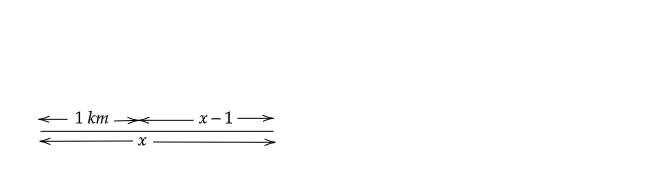# A three-wheeler scooter charges Rs. 15 for first kilometer and Rs. 8 each for every subsequent kilometer. For a distance of $x\ km$, an amount of Rs. $y$ is paid. Write the linear equation representing the above information.

Given:

A three-wheeler scooter charges Rs. 15 for first kilometer and Rs. 8 each for every subsequent kilometre. For a distance of $x\ km$, an amount of Rs. $y$ is paid.

To do:

We have to write the linear equation representing the above information.

Solution:

Let the total distance covered be $x\ km$.

Fare for the first kilometer $=1\times 15=Rs.\ 15$Fare for the subsequent distance$=Rs.\ ( x-1)\times8$

According to the question,

$15+( x-1)8=y$

$\Rightarrow 15+8x-8=y$

$\Rightarrow 8x-y+7=0$

The linear equation representing the given information is $8x-y+7=0$.

Updated on: 10-Oct-2022

68 Views